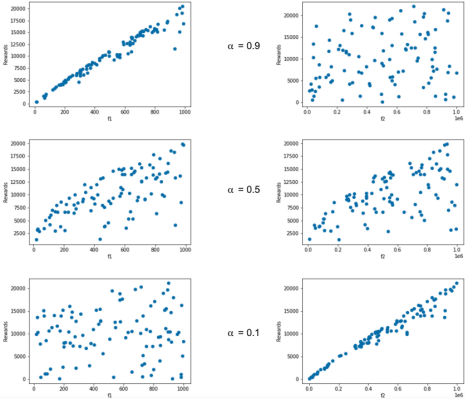# Activity Rewards (Under Development)

For each epoch (4 weeks), there will be a pool alloted for rewards, R, to be split between all Curators, Collectors and Creators. Each indibidual in the pool will receive an individual attention score, w, that will be used to determine the reward, r, for a specific individual. The individual score is determined by the formula
$w = f^α_1 \times f_2^{1-\alpha}$
where
$f_1$
is a quantifiable good behaviour factor and
$f_2$
is the second good behaviour factor for this individual category over that period.
Alpha (α) is a constant that allows increased or decreased weighting on either on either
$f_1$
or
$f_2$
and this is demonstrated through the "Rewards of
$f_1$
and
$f_2$
" plots at the end of this section. Changing
$\alpha$
over a period of time allows TokenTraxx to manage the goals of the platform through the incentive mechanism for users.
For example, let's say in the beginnning of protocol, we want to focus on "Democratisation over revenue generation".
Using the
$f_1$
and
$f_2$
for Creators in the table below, we can initially set
$\alpha = 0.9$
to reward Creators to increase "# of listeners/epoch" when they launch a campaign. We can then gradually decrease
$\alpha$
to 0.1 in the future epochs, which will then reward Creators who have generated the highest revenue for the protocol, thus incentivising the highest monetary value generator Creators with the best music.
Individual Score Formula
$w = f^α_1 \times f_2^{1-\alpha}$
Individual Reward Formula
$r = R \times \cfrac{w}{{\displaystyle\sum_{n}^k}w_n} , n = 1,2... k$
Variable
Definition
$w$
Individual Score
$f_1$
Good Behaviour Factor #1
$f_2$
Good Behaviour Factor #2
$\alpha$
Constant, determines weighting of
$f_1$
vs
$f_2$
$\downarrow\alpha\rArr \uparrow f_2 \downarrow f_1$
$\uparrow\alpha\rArr\downarrow f_2 \uparrow f_1$
Initial Value = 0.9, see reward plots
$r$
Reward for individual
R
Total reward pool for epoch
${{\displaystyle\sum_{n}^k}w_n}$
Sum of scores for everyone within a specific category (Creator/Curator/Collector)
Examples of Good Behaviour Factors
Text
Creators
Curators
Collectors
$f_1$
High # of listeners/epoch
High # of curated playlists
High Avg. Listens/Day
$f_2$
Revenue generated for the protocol
High total subscribed collectors
High Token spend on artist
Rewards of
$f_1$
and
$f_2$
for
$\alpha=0.9,0.5^*,0.1^*$
*other values of
$\alpha$
shown to display weight effect of
$f_1$
vs
$f_2$